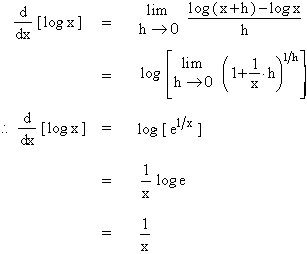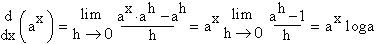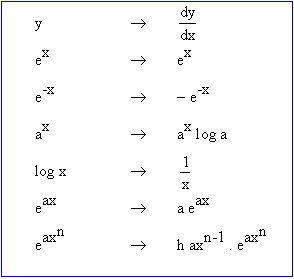Home MonkeyNotes Printable Notes Digital Library Study Guides Study Smart Parents Tips College Planning Test Prep Fun Zone Help / FAQ How to Cite New Title Request

From the definition of the derivatives, we getDifferentiation of the general exponential function ax, a > 0

Let y = ax i.e. f ( x ) = ax Þ f ( x + h ) = ax+h = ax . ah .

From the definition of the derivative, we getYour browser does not support the IFRAME tag.

Thus we have,Index

4. 1 Derivability At A Point
4. 2 Derivability In An Interval
4. 3 Derivability And Continuity Of A Function At A Point
4. 4 Some Counter Examples

4. 5 Interpretation Of Derivatives
4. 6 Theorems On Derivatives (differentiation Rules)
4. 7 Derivatives Of Standard Functions
4. 8 Derivative Of A Composite Function
4. 9 Differentiation Of Implicit Functions
4.10 Derivative Of An Inverse Function
4.11 Derivatives Of Inverse Trigonometric Functions
4.12 Derivatives Of Exponential & Logarithmic Functions
4.13 Logarithmic Differentiation
4.14 Derivatives Of Functions In Parametric Form
4.15 Higher order Derivatives

Chapter 5All Contents Copyright © All rights reserved.
Further Distribution Is Strictly Prohibited.

1210146 PinkMonkey users are on the site and studying right now.